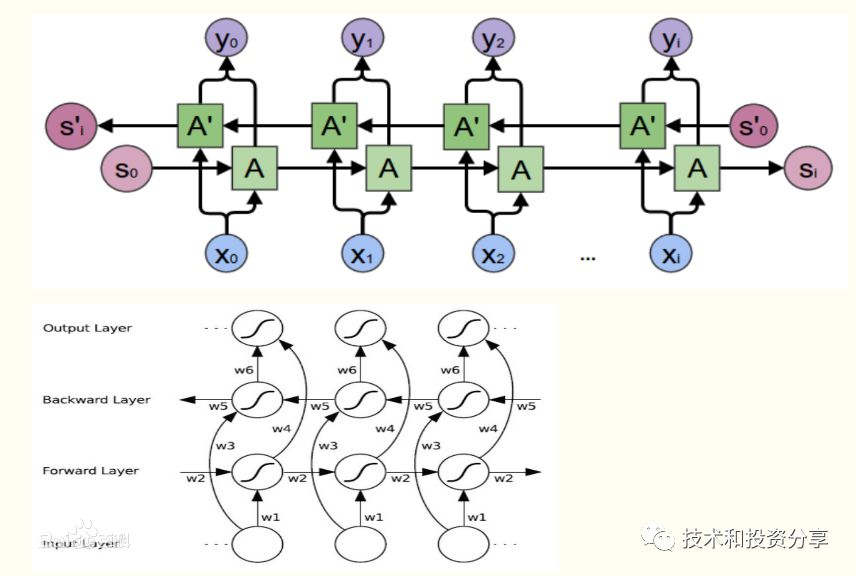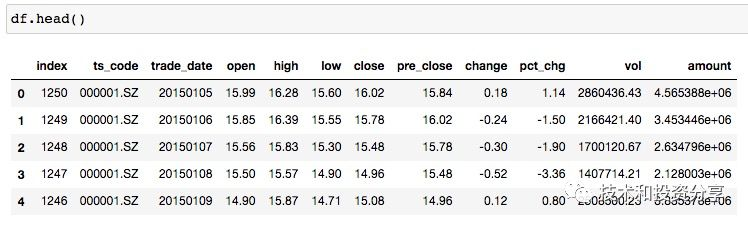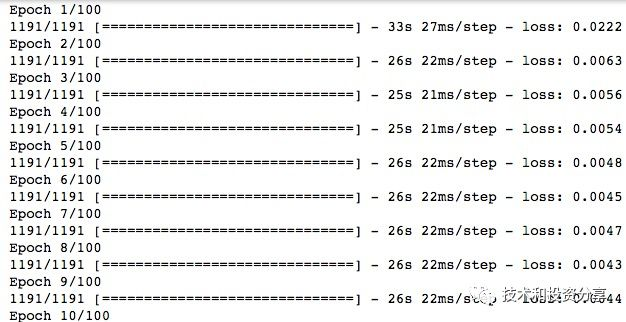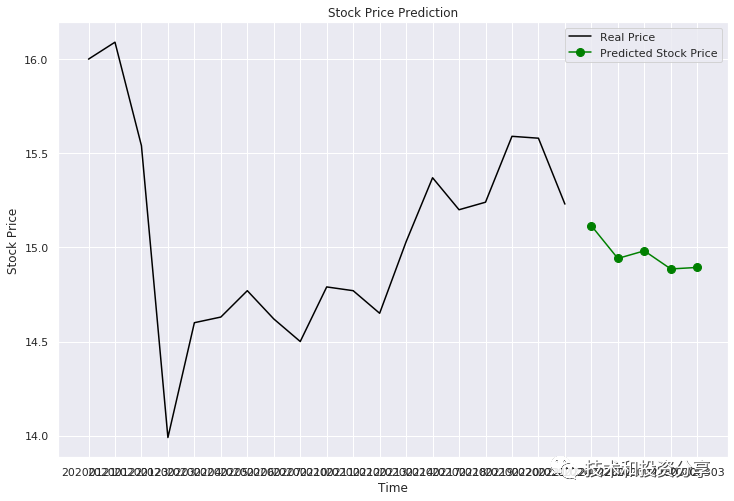# 用深度学习算法预测未来股票走势1.引入需要的包

 1 2 3 4  import numpy as np import pandas as pd import matplotlib.pyplot as plt import tushare as ts #我们是使用 tushare 来下载股票数据 

2.下载股票数据

 1 2 3 4 5 6 7  ts.set_token('xxx') #需要在 tushare 官网申请一个账号，然后得到 token 后才能通过数据接口获取数据 pro = ts.pro_api() #这里是用 000001 平安银行为例，下载从 2015-1-1 到最近某一天的股价数据 df = pro.daily(ts_code=‘000001.SZ’, start_date=‘2015-01-01’, end_date=‘2020-02-25’) df.head() #用 df.head() 可以查看一下下载下来的股票价格数据，显示数据如下：3.做数据准备

 1 2 3 4 5 6 7 8 9 10 11 12 13 14 15 16 17 18 19 20 21 22 23  #把数据按时间调转顺序，最新的放后面，从 tushare 下载的数据是最新的在前面，为了后面准备 X,y 数据方便 df = df.iloc[::-1] df.reset_index(inplace=True) #只用数据里面的收盘价字段的数据，也可以测试用更多价格字段作为预测输入数据 training_set = df.loc[:, ['close']] #只取价格数据，不要表头等内容 training_set = training_set.values #对数据做规则化处理，都按比例转成 0 到 1 之间的数据，这是为了避免真实数据过大或过小影响模型判断 from sklearn.preprocessing import MinMaxScaler sc = MinMaxScaler(feature_range = (0, 1)) training_set_scaled = sc.fit_transform(training_set) #准备 X 和 y 数据，就类似前面解释的，先用最近一个交易日的收盘价作为第一个 y，然后这个交易日以前的 60 个交易日的收盘价作为 X。 #这样依次往前推，例如最近第二个收盘价是第二个 y，而最新第二个收盘价以前的 60 个交易日收盘价作为第二个 X，依次往前准备出大量的 X 和 y，用于后面的训练。 X_train = [] y_train = [] for i in range(60, len(training_set_scaled)): X_train.append(training_set_scaled[i-60:i]) y_train.append(training_set_scaled[i, training_set_scaled.shape - 1]) X_train, y_train = np.array(X_train), np.array(y_train) 

4.创建 LSTM 模型并训练

 1 2 3 4 5 6 7 8 9 10 11 12 13 14 15 16 17 18 19 20 21 22 23 24 25 26  #这里是使用 Keras，Keras 大大简化了模型创建工作，背后的真正算法实现是用 TensorFlow 或其他。 from keras.models import Sequential from keras.layers import Dense from keras.layers import LSTM from keras.layers import Dropout regressor = Sequential() regressor.add(LSTM(units = 50, return_sequences = True, input_shape = (X_train.shape, X_train.shape))) regressor.add(Dropout(0.2)) regressor.add(LSTM(units = 50, return_sequences = True)) regressor.add(Dropout(0.2)) regressor.add(LSTM(units = 50, return_sequences = True)) regressor.add(Dropout(0.2)) regressor.add(LSTM(units = 50)) regressor.add(Dropout(0.2)) regressor.add(Dense(units = 1)) regressor.compile(optimizer = 'adam', loss = 'mean_squared_error') regressor.fit(X_train, y_train, epochs = 100, batch_size = 32)5.预测未来的价格

 1 2 3 4 5 6 7 8 9 10 11 12 13 14 15 16 17 18 19 20 21 22 23 24 25 26 27 28 29 30 31 32 33 34 35 36 37 38 39 40 41 42 43  import tushare as ts ts.set_token('xxx') pro = ts.pro_api() df_test = pro.index_daily(ts_code='000001.SZ', start_date='2020-02-26', end_date='2020-02-26') #也是把数据调转顺序，最新的放后面 df_test = df_test.iloc[::-1] df_test.reset_index(inplace=True) #只用 close 收盘价这个字段 dataset_test = df_test.loc[:, ['close']] #然后把测试数据和前面的训练数据整合到一起 dataset_total = pd.concat( (df_test[['close']]，df[['close']]), axis = 0) #也是只取具体数值，去掉表头等信息 inputs = dataset_total[len(dataset_total) - len(dataset_test) - 60:].values #这里要按照特定的格式要求做一个数组变形，Keras 对数据格式有特定要求 inputs = inputs.reshape(-1, dataset_test.shape) #对数据也要做一次规则化处理 inputs = sc.transform(inputs) predicted_stock_price = [] #准备测试数据，就是把要测试的数据和以前训练的数据结合起来组装出要测试的 X，因为是要利用过去 60 个交易日的数据，只靠一个交易日的收盘价是不够的 X_test = [] for i in range(60, 60 + len(dataset_test)): X_test.append(inputs[i-60:i]) X_test = np.array(X_test) #对预测数据也做一次数组变形处理 X_test = np.reshape(X_test, (X_test.shape, X_test.shape, dataset_test.shape)) #用前面训练的模型预测价格，得出来的是从 0 到 1 之间的规则化数值 predicted_stock_price = regressor.predict(X_test) #再把规则化数据转回成正常的价格数据，现在就可以得出预测的下个交易日收盘价格 predicted_stock_price = sc.inverse_transform(predicted_stock_price)10-28
12-235万+01-042万+
11-122万+
01-141075
10-291262
06-014万+
09-11